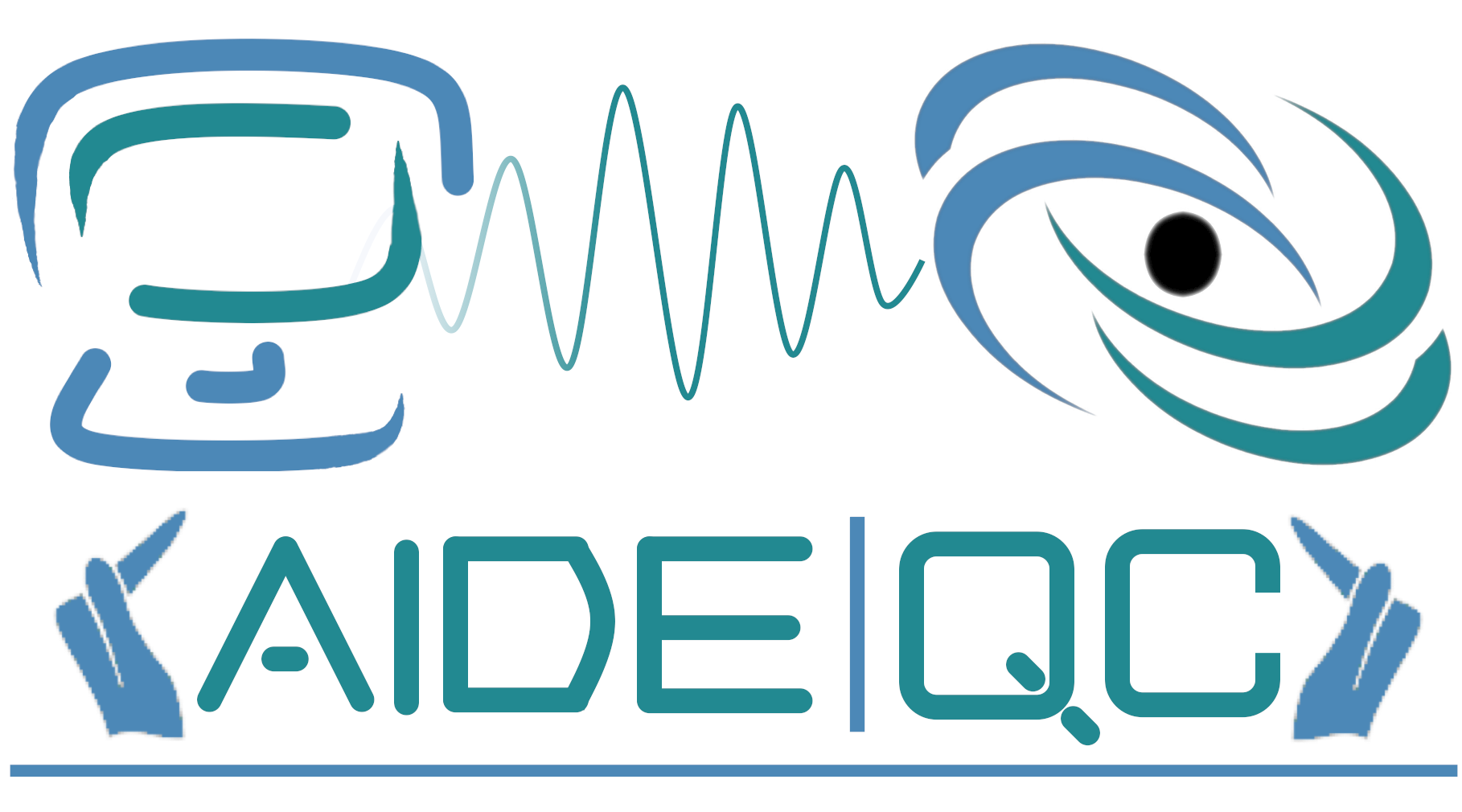#AIDE-QC

Advanced Integrated Development Environment for Quantum Computing

# Execution Model

The QCOR execution model considers a hybrid quantum-classical compute system that enables execution of quantum kernels on available quantum coprocessors in a quantum-hardware agnostic fashion. The host-side, classical execution model should simply be the execution model of the language being extended (e.g. C++, Python, etc.).

QCOR treats the quantum coprocessors as an abstract machine that loads and executes general host-specified quantum instructions and persists measurement results (measured classical bits) to a quantum-processor-local discrete memory space, with synchronization of that memory space with the host-side handled implicitly by language extension implementations. Each specified quantum instruction for execution carries with it information about its unique instruction identifier, the qubit to operate upon (the index of the qubit in the infinitely-sized, assumed global qubit register, see Memory Model ), and any required or extra metadata about the operation. Quantum instructions loaded for execution can be single instructions or a composite of single instructions, thereby enabling one to submit, load, and execute entire quantum circuits or programs.

This latter point enables the QCOR execution model to retain a multi-modal characteristic. The execution model should enable both near-term, loosely-coupled (remotely-hosted) batch circuit execution models (NISQ mode execution), as well as future, tightly integrated CPU-QPU architectures with fast-feedback (FTQC mode execution, fault-tolerant quantum computing). FTQC mode execution streams quantum instruction executions, i.e. as the program is running, invoked quantum instructions will affect the qubit register as soon as they are encountered. NISQ mode execution should instead queue encountered quantum instructions and flush the queue upon exiting an entry-point quantum kernel.

Language implementations should enable both modes of execution, and this implies additional programming capabilities that may exist in one mode over the other. For example, FTQC mode execution should enable programmers to leverage the classical language control flow structures enabling fast-feedback on qubit measurement results.

Here are two examples showing the differences in programming for NISQ and FTQC mode execution (in a C++ QCOR language extension). First we show FTQC mode execution, where one can clearly see the ability to stream quantum instruction invocations, followed by conditional statements on qubit measurement results.

``````__qpu__ void PrepareStateUsingRUS(qreg q, int maxIter) {
// Note: target = q, aux = q
auto r = q.tail();
H(r);
// We limit the max number of RUS iterations.
for (int i = 0; i < maxIter; ++i) {
Tdg(r);
X::ctrl(q, r);
T(r);

// In order to measure in the PauliX basis, changes the basis.
H(r);
if (!Measure(r)) {
// Success (until (outcome == Zero))
std::cout << "Success after " << i + 1 << " iterations.\n";
break;
}
else {
// Measure 1: |1> state
// Fix up: Bring the auxiliary and target qubits back to |+> state.
X(r);
H(r);
X(q);
H(q);
}
}
}
``````

Next, we show the construction of a QAOA ansatz on a general cost Hamiltonian. We still get the usual control flow (`for` loops, etc.), but the circuit is in a sense constructed by this quantum kernel before ultimate execution on the loosely-coupled quantum processor. The stream of instructions is implicitly flushed (executed) upon exiting this entry-point quantum kernel.

``````__qpu__ void qaoa_ansatz(qreg q, int n_steps, std::vector<double> gamma,
std::vector<double> beta, Operator cost_ham) {

// Local Declarations
auto nQubits = q.size();
int gamma_counter = 0;
int beta_counter = 0;

// Start of in the uniform superposition
H(q);

// Get all non-identity hamiltonian terms
// for the following exp(H_i) trotterization
auto cost_terms = cost_ham.getNonIdentitySubTerms();

// Loop over qaoa steps
for (int step = 0; step < n_steps; step++) {

// Loop over cost hamiltonian terms
for (int i = 0; i < cost_terms.size(); i++) {

auto cost_term = cost_terms[i];
auto m_gamma = gamma[gamma_counter];

// trotterize
exp_i_theta(q, m_gamma, cost_term);

gamma_counter++;
}

// Add the reference hamiltonian term
for (int i = 0; i < nQubits; i++) {
auto ref_ham_term = X(i);
auto m_beta = beta[beta_counter];
exp_i_theta(q, m_beta, ref_ham_term);
beta_counter++;
}
}
}
``````